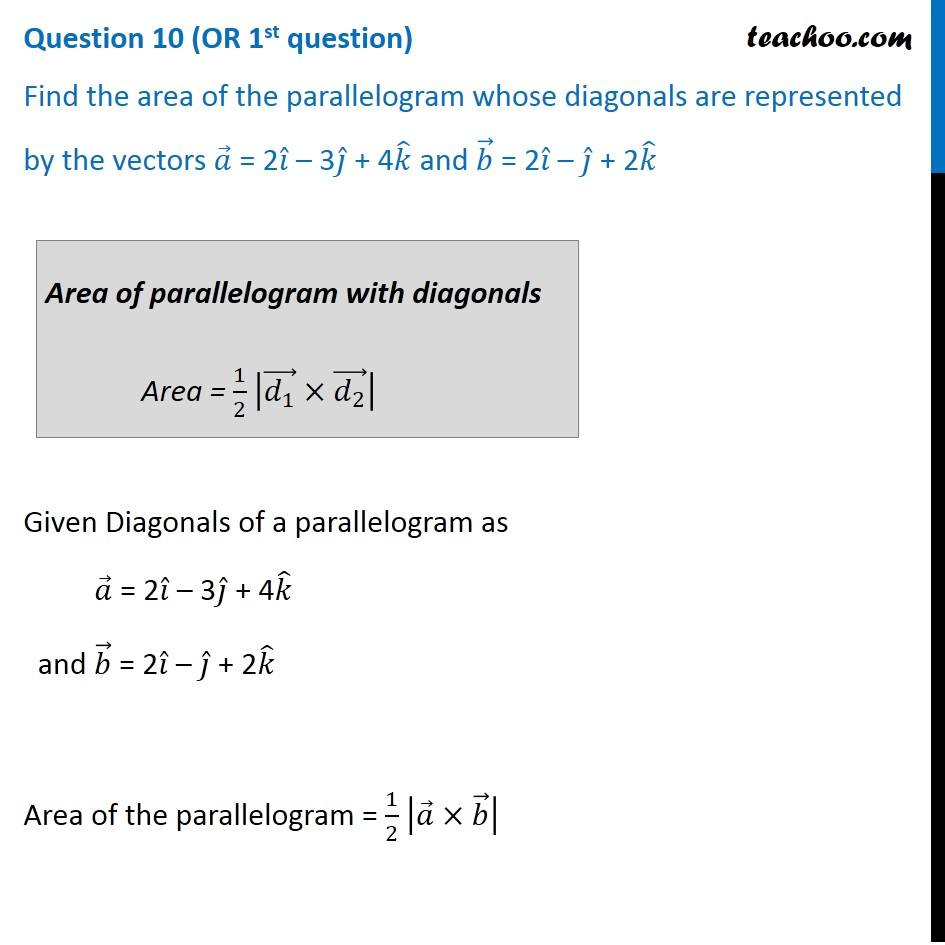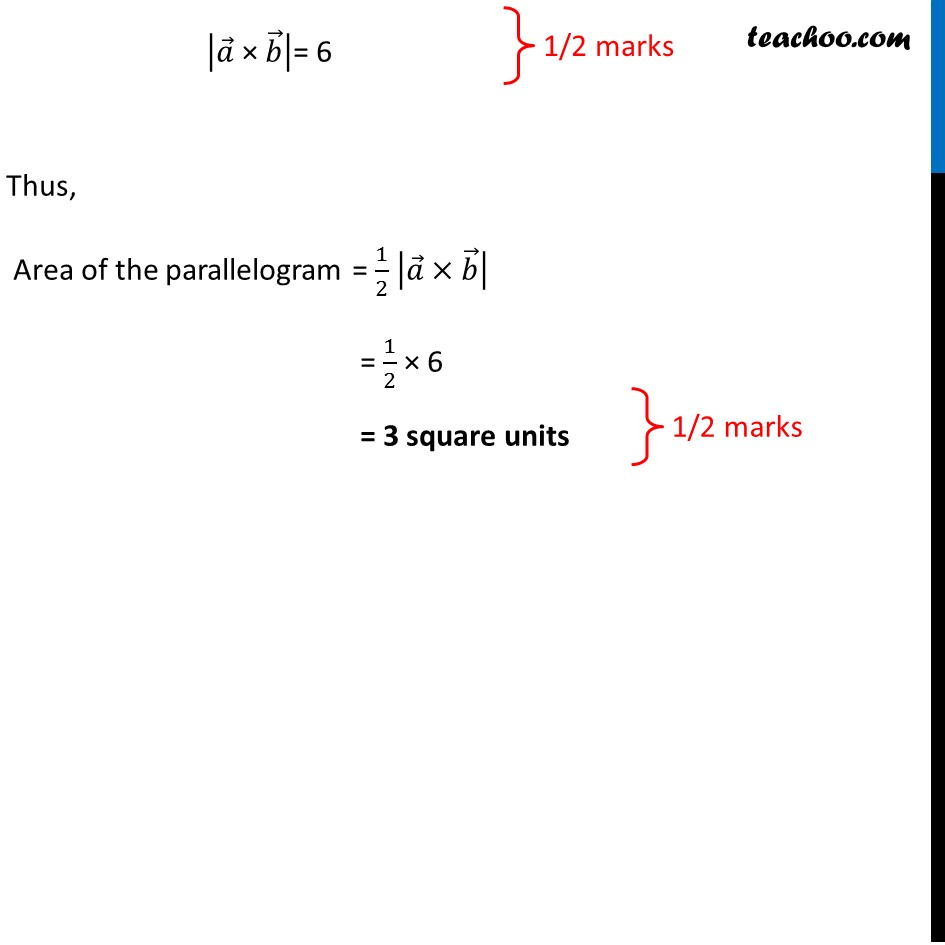CBSE Class 12 Sample Paper for 2019 Boards

Class 12
Solutions of Sample Papers and Past Year Papers - for Class 12 Boards

Question 10 (OR 1 st question)

Find the area of the parallelogram whose diagonals are represented by the vectors a = 2i  – 3j  + 4k and b = 2i – j + 2kLearn in your speed, with individual attention - Teachoo Maths 1-on-1 Class

### Transcript

Question 10 (OR 1st question) Find the area of the parallelogram whose diagonals are represented by the vectors 𝑎 ⃗ = 2𝑖 ̂ – 3𝑗 ̂ + 4𝑘 ̂ and 𝑏 ⃗ = 2𝑖 ̂ – 𝑗 ̂ + 2𝑘 ̂ Area of parallelogram with diagonals Area = 1/2 |(𝑑_1 ) ⃗×(𝑑_2 ) ⃗ | Given Diagonals of a parallelogram as 𝑎 ⃗ = 2𝑖 ̂ – 3𝑗 ̂ + 4𝑘 ̂ and 𝑏 ⃗ = 2𝑖 ̂ – 𝑗 ̂ + 2𝑘 ̂ Area of the parallelogram = 1/2 |𝑎 ⃗×𝑏 ⃗ | Finding 𝒂 ⃗ × 𝒃 ⃗ 𝑎 ⃗ × 𝑏 ⃗ = |■8(𝑖 ̂&𝑗 ̂&𝑘 ̂@2&−3&4@2&−1&2)| = 𝑖 ̂ ((–3) × 2 – (-1) × 4) − 𝑗 ̂ (2 × 2 − 2 × 4) + 𝑘 ̂ (2 × (-1) − 2 × (-3)) = 𝑖 ̂ (-6 + 4) − 𝑗 ̂ (4 – 8) + 𝑘 ̂ (–2 + 6) = 𝑖 ̂ (−2) - 𝑗 ̂ (−4) + 𝑘 ̂ (4) = –2𝑖 ̂ + 4𝑗 ̂ + 4𝑘 ̂ So, Magnitude of 𝑎 ⃗ × 𝑏 ⃗ = √((−2)2+(4)2+(4)2) |𝑎 ⃗" × " 𝑏 ⃗ | = √(4+16+16) |𝑎 ⃗" × " 𝑏 ⃗ |= √36 |𝑎 ⃗" × " 𝑏 ⃗ |= 6 Thus, Area of the parallelogram = 1/2 |𝑎 ⃗×𝑏 ⃗ | = 1/2 × 6 = 3 square units